# NCERT Exemplar Class 12 Physics Solutions for Chapter 6 Electromagnetic Induction

The NCERT Class 12 Physics Exemplar for Chapter 6 Electromagnetic Induction consists of different kinds of questions and answers to the questions given in the NCERT Exemplar Class 12 Physics book. Together with textbook questions, BYJU’S Exemplar for Class 12 comprises extra questions, numericals, fill in the blanks, MCQs and short answer questions.

The NCERT Exemplar Class 12 Physics book is a vital study material for students who are preparing for the Class 12 board examination and national-level graduate entrance examination. Exemplar problems help you understand topics clearly.

## Download the PDF of NCERT Exemplar for Class 12 Physics Chapter 6 Electromagnetic Induction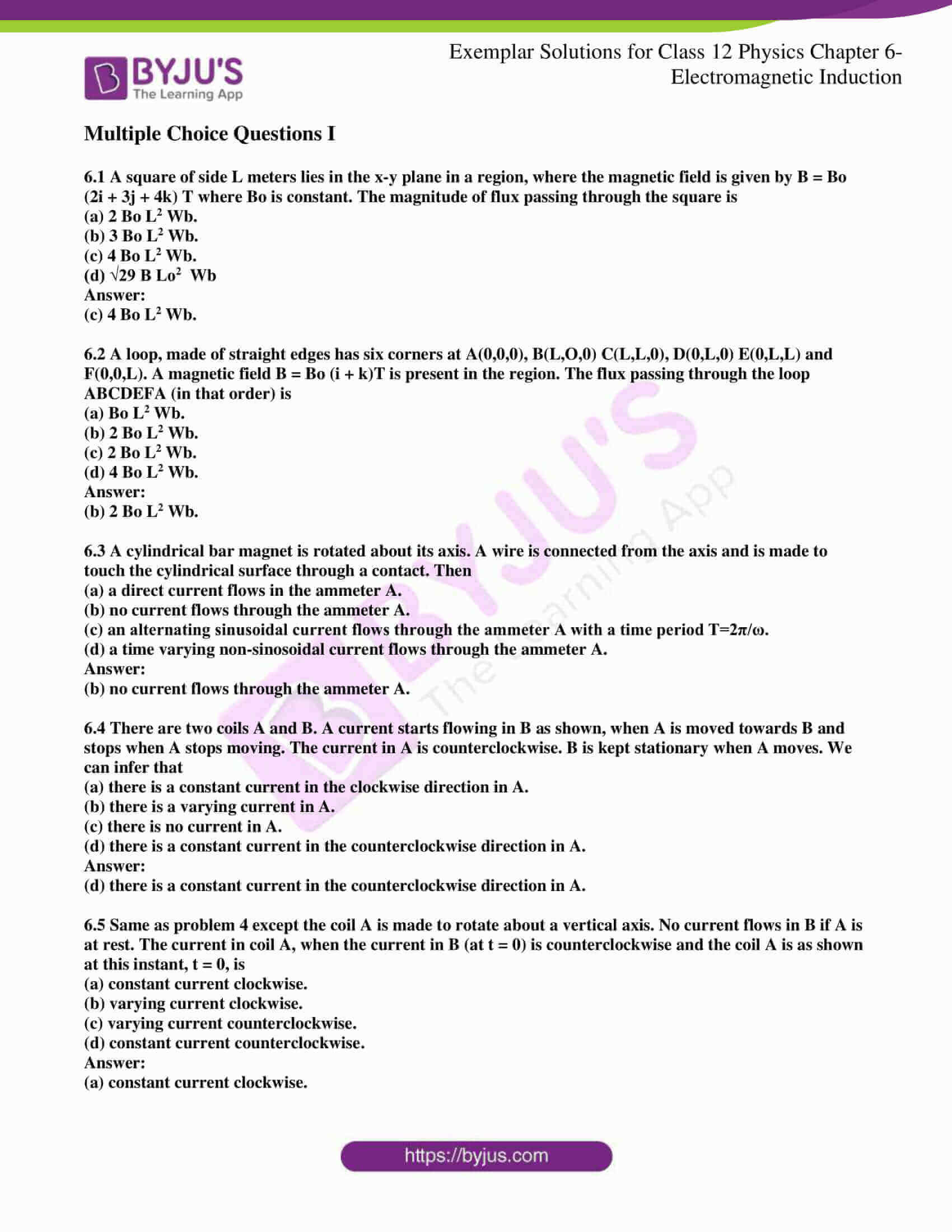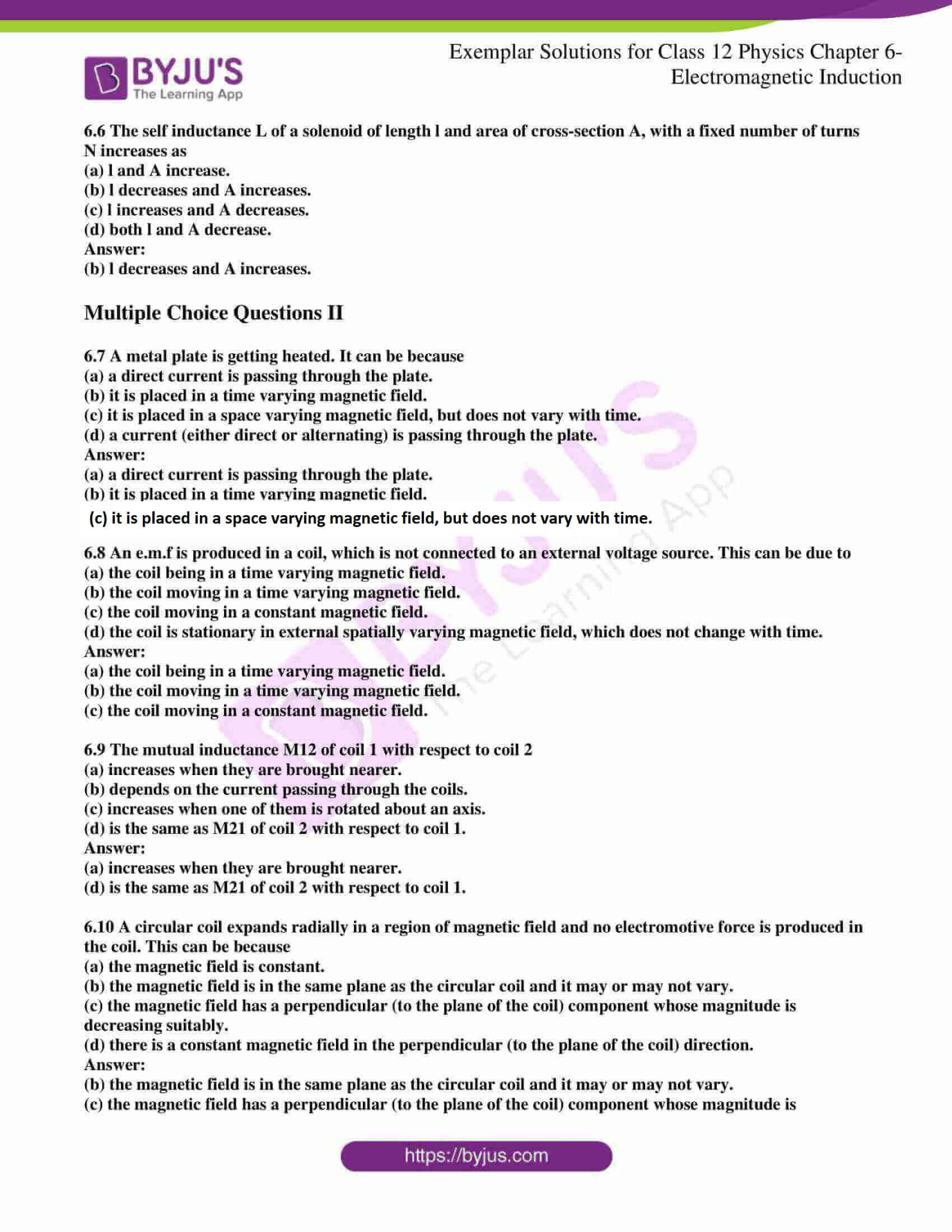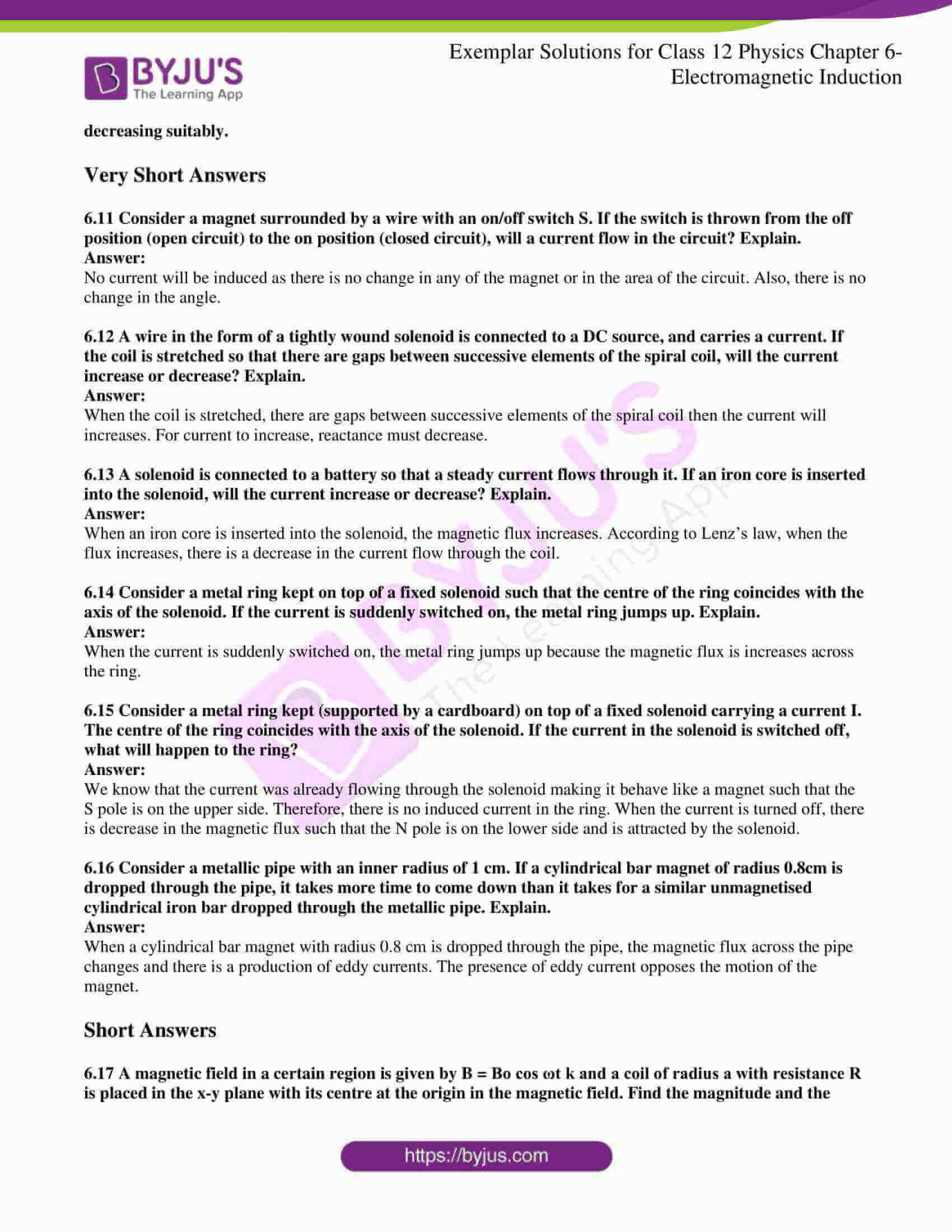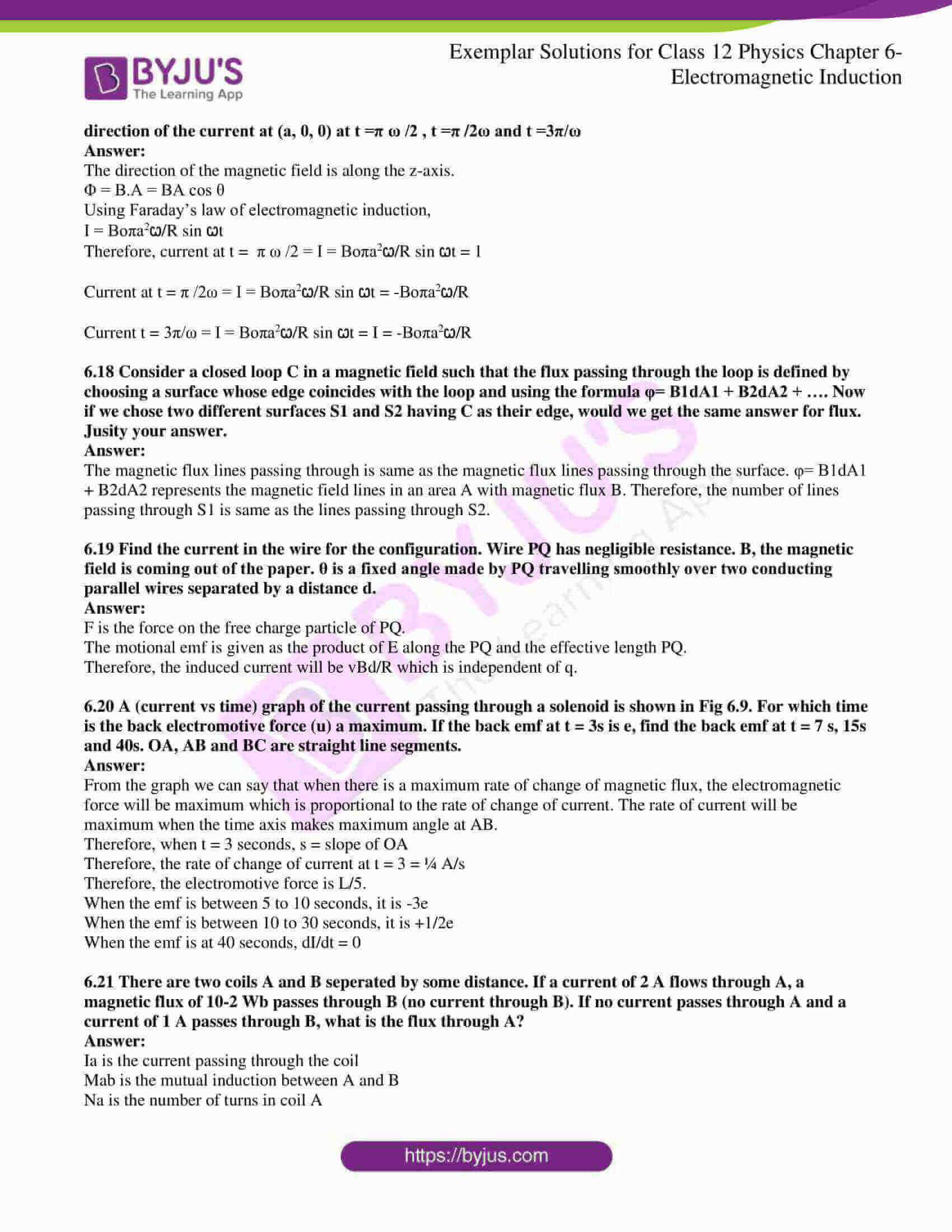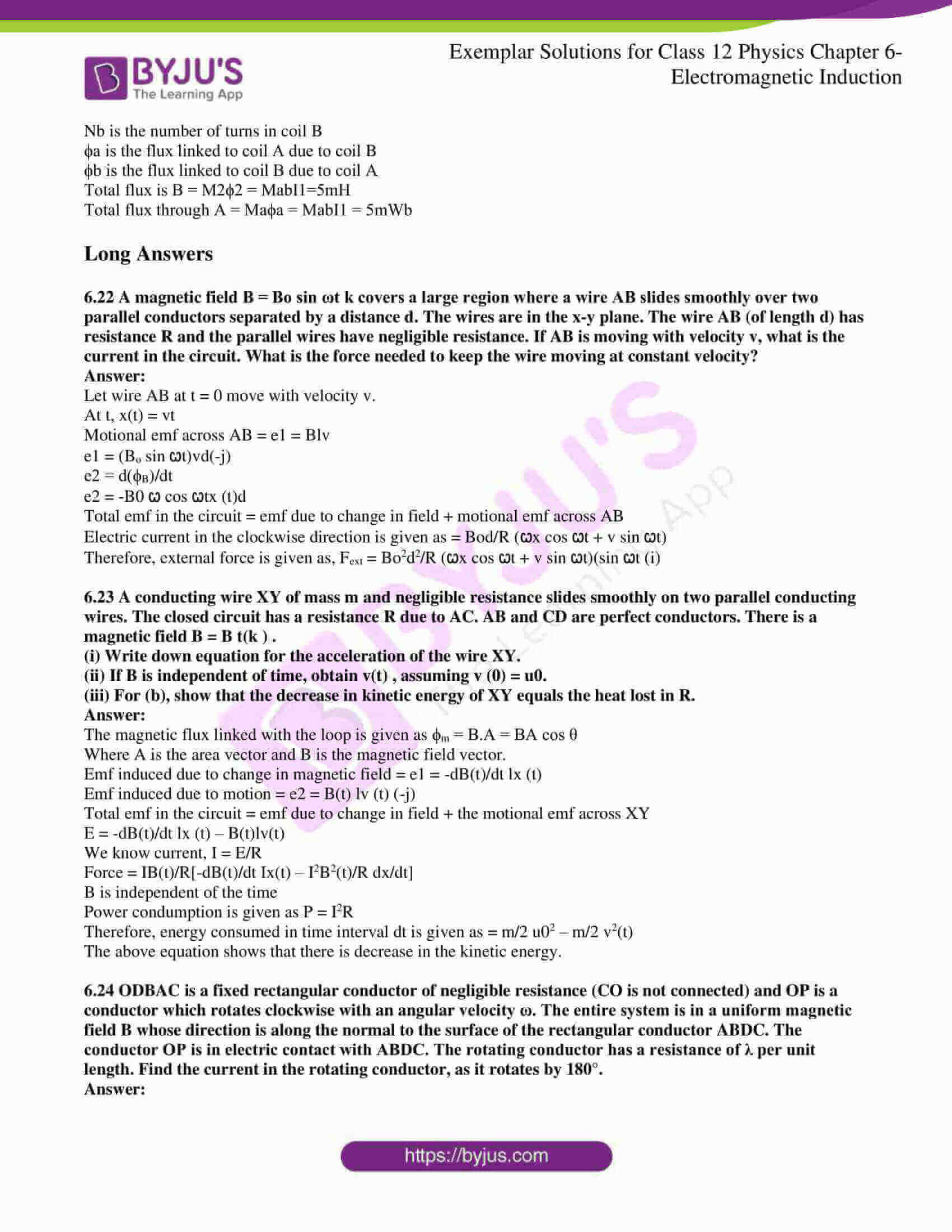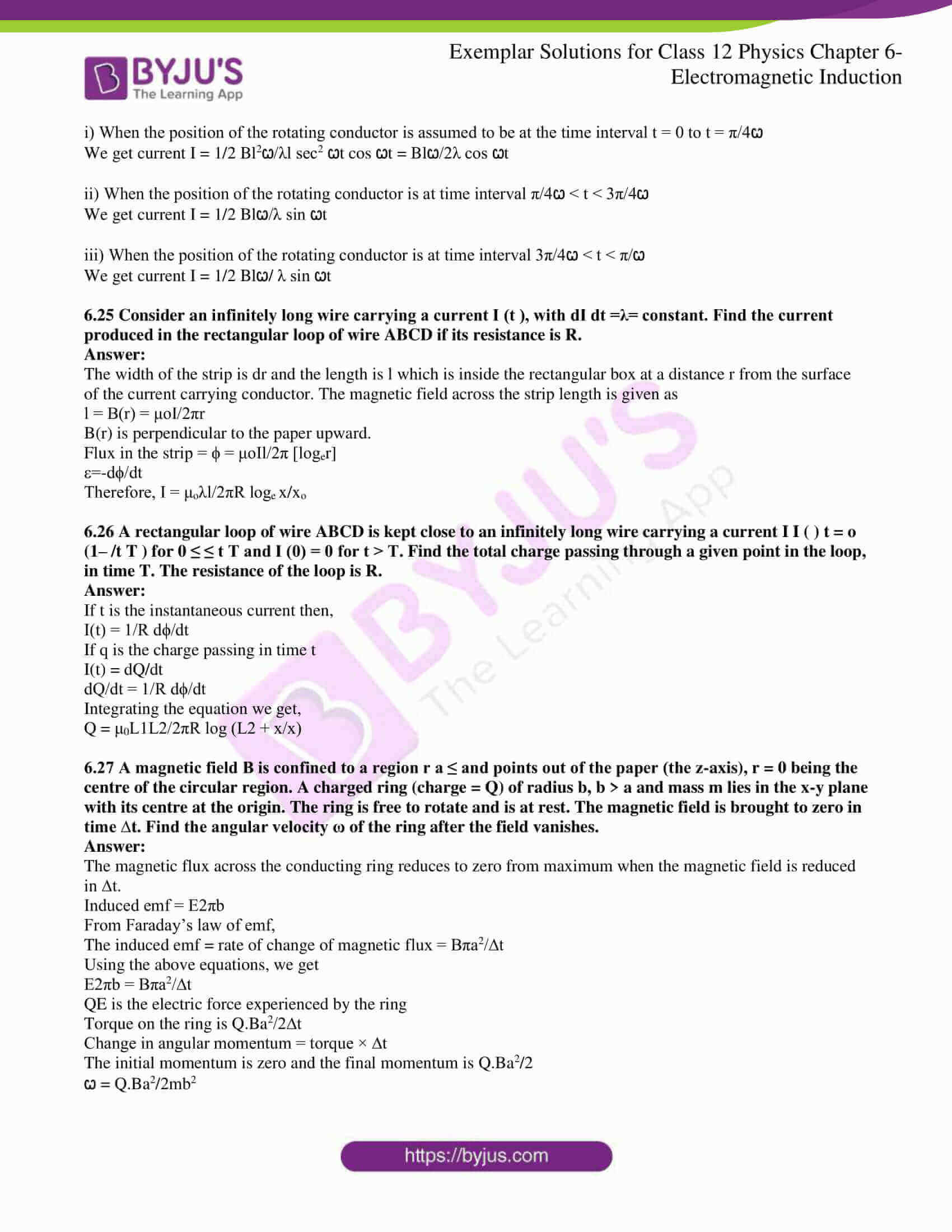### Multiple Choice Questions I

6.1 A square of side L meters lies in the x-y plane in a region, where the magnetic field is given by B = Bo (2i + 3j + 4k) T where Bo is constant. The magnitude of flux passing through the square is

(a) 2 Bo L2 Wb.

(b) 3 Bo L2 Wb.

(c) 4 Bo L2 Wb.

(d) √29 B Lo2 Wb

(c) 4 Bo L2 Wb.

6.2 A loop, made of straight edges has six corners at A(0,0,0), B(L,O,0) C(L,L,0), D(0,L,0) E(0,L,L) and F(0,0,L). A magnetic field B = Bo (i + k)T is present in the region. The flux passing through the loop ABCDEFA (in that order) is

(a) Bo L2 Wb.

(b) 2 Bo L2 Wb.

(c) 2 Bo L2 Wb.

(d) 4 Bo L2 Wb.

(b) 2 Bo L2 Wb.

6.3 A cylindrical bar magnet is rotated about its axis. A wire is connected from the axis and is made to touch the cylindrical surface through a contact. Then

(a) a direct current flows in the ammeter A.

(b) no current flows through the ammeter A.

(c) an alternating sinusoidal current flows through the ammeter A with a time period T=2π/ω.

(d) a time-varying non-sinusoidal current flows through the ammeter A.

(b) no current flows through the ammeter A.

6.4 There are two coils A and B. A current starts flowing in B as shown, when A is moved towards B and stops when A stops moving. The current in A is counterclockwise. B is kept stationary when A moves. We can infer that

(a) there is a constant current in the clockwise direction in A.

(b) there is a varying current in A.

(c) there is no current in A.

(d) there is a constant current in the counterclockwise direction in A.

(d) there is a constant current in the counterclockwise direction in A.

6.5 Same as problem 4 except the coil A is made to rotate about a vertical axis. No current flows in B if A is at rest. The current in coil A, when the current in B (at t = 0) is counterclockwise and the coil A is as shown at this instant, t = 0, is

(a) constant current clockwise.

(b) varying current clockwise.

(c) varying current counterclockwise.

(d) constant current counterclockwise.

(a) constant current clockwise.

6.6 The self-inductance L of a solenoid of length l and area of cross-section A, with a fixed number of turns N increases as

(a) l and A increase.

(b) l decreases and A increases.

(c) l increases and A decreases.

(d) both l and A decrease.

(b) l decreases and A increases.

### Multiple Choice Questions II

6.7 A metal plate is getting heated. It can be because

(a) a direct current is passing through the plate.

(b) it is placed in a time-varying magnetic field.

(c) it is placed in a space varying magnetic field, but does not vary with time.

(d) a current (either direct or alternating) is passing through the plate.

(a) a direct current is passing through the plate.

(b) it is placed in a time-varying magnetic field.

(c) it is placed in a space varying magnetic field, but does not vary with time.

6.8 An e.m.f is produced in a coil, which is not connected to an external voltage source. This can be due to

(a) the coil is in a time-varying magnetic field.

(b) the coil moving in a time-varying magnetic field.

(c) the coil moving in a constant magnetic field.

(d) the coil is stationary in an external spatially varying magnetic field, which does not change with time.

(a) the coil is in a time-varying magnetic field.

(b) the coil moving in a time-varying magnetic field.

(c) the coil moving in a constant magnetic field.

6.9 The mutual inductance M12 of coil 1 with respect to coil 2

(a) increases when they are brought nearer.

(b) depends on the current passing through the coils.

(c) increases when one of them is rotated about an axis.

(d) is the same as M21 of coil 2 with respect to coil 1.

(a) increases when they are brought nearer.

(d) is the same as M21 of coil 2 with respect to coil 1.

6.10 A circular coil expands radially in a region of the magnetic field and no electromotive force is produced in the coil. This can be because

(a) the magnetic field is constant.

(b) the magnetic field is in the same plane as the circular coil and it may or may not vary.

(c) the magnetic field has a perpendicular (to the plane of the coil) component whose magnitude is decreasing suitably.

(d) there is a constant magnetic field in the perpendicular (to the plane of the coil) direction.

(b) the magnetic field is in the same plane as the circular coil and it may or may not vary.

(c) the magnetic field has a perpendicular (to the plane of the coil) component whose magnitude is decreasing suitably.

6.11 Consider a magnet surrounded by a wire with an on/off switch S. If the switch is thrown from the off position (open circuit) to the on position (closed circuit), will a current flow in the circuit? Explain.

No current will be induced as there is no change in any of the magnet or in the area of the circuit. Also, there is no change in the angle.

6.12 A wire in the form of a tightly wound solenoid is connected to a DC source, and carries a current. If the coil is stretched so that there are gaps between successive elements of the spiral coil, will the current increase or decrease? Explain.

When the coil is stretched, there are gaps between successive elements of the spiral coil then the current will increases. For current to increase, reactance must decrease.

6.13 A solenoid is connected to a battery so that a steady current flows through it. If an iron core is inserted into the solenoid, will the current increase or decrease? Explain.

When an iron core is inserted into the solenoid, the magnetic flux increases. According to Lenz’s law, when the flux increases, there is a decrease in the current flow through the coil.

6.14 Consider a metal ring kept on top of a fixed solenoid such that the centre of the ring coincides with the axis of the solenoid. If the current is suddenly switched on, the metal ring jumps up. Explain.

When the current is suddenly switched on, the metal ring jumps up because the magnetic flux is increased across the ring.

6.15 Consider a metal ring kept (supported by a cardboard) on top of a fixed solenoid carrying a current I. The centre of the ring coincides with the axis of the solenoid. If the current in the solenoid is switched off, what will happen to the ring?

We know that the current was already flowing through the solenoid making it behave like a magnet such that the S pole is on the upper side. Therefore, there is no induced current in the ring. When the current is turned off, there is a decrease in the magnetic flux such that the N pole is on the lower side and is attracted by the solenoid.

6.16 Consider a metallic pipe with an inner radius of 1 cm. If a cylindrical bar magnet of radius 0.8cm is dropped through the pipe, it takes more time to come down than it takes for a similar unmagnetised cylindrical iron bar dropped through the metallic pipe. Explain.

When a cylindrical bar magnet with radius 0.8 cm is dropped through the pipe, the magnetic flux across the pipe changes and there is a production of eddy currents. The presence of eddy current opposes the motion of the magnet.

6.17 A magnetic field in a certain region is given by B = Bo cos ωt k and a coil of radius a with resistance R is placed in the x-y plane with its centre at the origin in the magnetic field. Find the magnitude and the direction of the current at (a, 0, 0) at t =π ω /2, t =π /2ω and t =3π/ω

The direction of the magnetic field is along the z-axis.

Φ = B.A = BA cos θ

Using Faraday’s law of electromagnetic induction,

I = Boπa2ꞷ/R sin ꞷt

Therefore, current at t = π ω /2 = I = Boπa2ꞷ/R sin ꞷt = 1

Current at t = π /2ω = I = Boπa2ꞷ/R sin ꞷt = -Boπa2ꞷ/R

Current t = 3π/ω = I = Boπa2ꞷ/R sin ꞷt = I = -Boπa2ꞷ/R

6.18 Consider a closed loop C in a magnetic field such that the flux passing through the loop is defined by choosing a surface whose edge coincides with the loop and using the formula φ= B1dA1 + B2dA2 + …. Now if we chose two different surfaces S1 and S2 having C as their edge, would we get the same answer for flux. Justify your answer.

The magnetic flux lines passing through is the same as the magnetic flux lines passing through the surface. φ= B1dA1 + B2dA2 represents the magnetic field lines in an area A with magnetic flux B. Therefore, the number of lines passing through S1 is the same as the lines passing through S2.

6.19 Find the current in the wire for the configuration. Wire PQ has negligible resistance. B, the magnetic field is coming out of the paper. θ is a fixed angle made by PQ travelling smoothly over two conducting parallel wires separated by a distance d.

F is the force on the free charge particle of PQ.

The motional emf is given as the product of E along the PQ and the effective length PQ.

Therefore, the induced current will be vBd/R which is independent of q.

6.20 A (current vs time) graph of the current passing through a solenoid is shown in Fig 6.9. For which time is the back electromotive force (u) a maximum. If the back emf at t = 3s is e, find the back emf at t = 7 s, 15s and 40s. OA, AB and BC are straight line segments.

From the graph, we can say that when there is a maximum rate of change of magnetic flux, the electromagnetic force will be maximum which is proportional to the rate of change of current. The rate of current will be maximum when the time axis makes a maximum angle at AB.

Therefore, when t = 3 seconds, s = slope of OA

Therefore, the rate of change of current at t = 3 = ¼ A/s

Therefore, the electromotive force is L/5.

When the emf is between 5 to 10 seconds, it is -3e

When the emf is between 10 to 30 seconds, it is +1/2e

When the emf is at 40 seconds, dI/dt = 0

6.21 There are two coils A and B separated by some distance. If a current of 2 A flows through A, a magnetic flux of 10-2 Wb passes through B (no current through B). If no current passes through A and a current of 1 A passes through B, what is the flux through A?

Ia is the current passing through the coil

Mab is the mutual induction between A and B

Na is the number of turns in coil A

Nb is the number of turns in coil B

ϕa is the flux linked to coil A due to coil B

ϕb is the flux linked to coil B due to coil A

Total flux is B = M2ϕ2 = MabI1=5mH

Total flux through A = Maϕa = MabI1 = 5mWb

6.22 A magnetic field B = Bo sin ωt k covers a large region where a wire AB slides smoothly over two parallel conductors separated by a distance d. The wires are in the x-y plane. The wire AB (of length d) has resistance R and the parallel wires have negligible resistance. If AB is moving with velocity v, what is the current in the circuit? What is the force needed to keep the wire moving at constant velocity?

Let wire AB at t = 0 move with velocity v.

At t, x(t) = vt

Motional emf across AB = e1 = Blv

e1 = (Bo sin ꞷt)vd(-j)

e2 = d(ϕB)/dt

e2 = -B0 ꞷ cos ꞷtx (t)d

Total emf in the circuit = emf due to change in field + motional emf across AB

Electric current in the clockwise direction is given as = Bod/R (ꞷx cos ꞷt + v sin ꞷt)

Therefore, external force is given as, Fext = Bo2d2/R (ꞷx cos ꞷt + v sin ꞷt)(sin ꞷt (i)

6.23 A conducting wire XY of mass m and negligible resistance slides smoothly on two parallel conducting wires. The closed-circuit has a resistance R due to AC. AB and CD are perfect conductors. There is a magnetic field B = B t(k ).

(i) Write down the equation for the acceleration of the wire XY.

(ii) If B is independent of time, obtain v(t) , assuming v (0) = u0.

(iii) For (b), show that the decrease in kinetic energy of XY equals the heat lost in R.

The magnetic flux linked with the loop is given as ϕm = B.A = BA cos θ

Where A is the area vector and B is the magnetic field vector.

Emf induced due to change in magnetic field = e1 = -dB(t)/dt lx (t)

Emf induced due to motion = e2 = B(t) lv (t) (-j)

Total emf in the circuit = emf due to change in the field + the motional emf across XY

E = -dB(t)/dt lx (t) – B(t)lv(t)

We know current, I = E/R

Force = IB(t)/R[-dB(t)/dt Ix(t) – I2B2(t)/R dx/dt]

B is independent of the time

Power consumption is given as P = I2R

Therefore, energy consumed in time interval dt is given as = m/2 u02 – m/2 v2(t)

The above equation shows that there is a decrease in the kinetic energy.

6.24 ODBAC is a fixed rectangular conductor of negligible resistance (CO is not connected) and OP is a conductor which rotates clockwise with an angular velocity ω. The entire system is in a uniform magnetic field B whose direction is along the normal to the surface of the rectangular conductor ABDC. The conductor OP is in electric contact with ABDC. The rotating conductor has a resistance of λ per unit length. Find the current in the rotating conductor, as it rotates by 180°.

i) When the position of the rotating conductor is assumed to be at the time interval t = 0 to t = π/4ꞷ

We get current I = 1/2 Bl2ꞷ/λl sec2 ꞷt cos ꞷt = Blꞷ/2λ cos ꞷt

ii) When the position of the rotating conductor is at time interval π/4ꞷ < t < 3π/4ꞷ

We get current I = 1/2 Blꞷ/λ sin ꞷt

iii) When the position of the rotating conductor is at time interval 3π/4ꞷ < t < π/ꞷ

We get current I = 1/2 Blꞷ/ λ sin ꞷt

6.25 Consider an infinitely long wire carrying a current I (t ), with dI dt =λ= constant. Find the current produced in the rectangular loop of wire ABCD if its resistance is R.

The width of the strip is dr and the length is l which is inside the rectangular box at a distance r from the surface of the current-carrying conductor. The magnetic field across the strip length is given as

l = B(r) = μoI/2πr

B(r) is perpendicular to the paper upward.

Flux in the strip = ϕ = μoIl/2π [loger]

ε=-dϕ/dt

Therefore, I = μoλl/2πR loge x/xo

6.26 A rectangular loop of wire ABCD is kept close to an infinitely long wire carrying a current II ( ) t = o (1– /t T ) for 0 ≤ ≤ t T and I (0) = 0 for t > T. Find the total charge passing through a given point in the loop, in time T. The resistance of the loop is R.

If t is the instantaneous current then,

I(t) = 1/R dϕ/dt

If q is the charge passing in time t

I(t) = dQ/dt

dQ/dt = 1/R dϕ/dt

Integrating the equation we get,

Q = μ0L1L2/2πR log (L2 + x/x)

6.27 A magnetic field B is confined to a region r a ≤ and points out of the paper (the z-axis), r = 0 being the centre of the circular region. A charged ring (charge = Q) of radius b, b > a and mass m lies in the x-y plane with its centre at the origin. The ring is free to rotate and is at rest. The magnetic field is brought to zero in time ∆t. Find the angular velocity ω of the ring after the field vanishes.

The magnetic flux across the conducting ring reduces to zero from maximum when the magnetic field is reduced in ∆t.

Induced emf = E2πb

The induced emf = rate of change of magnetic flux = Bπa2/∆t

Using the above equations, we get

E2πb = Bπa2/∆t

QE is the electric force experienced by the ring

Torque on the ring is Q.Ba2/2∆t

Change in angular momentum = torque × ∆t

The initial momentum is zero and the final momentum is Q.Ba2/2

ꞷ = Q.Ba2/2mb2

6.28 A rod of mass m and resistance R slides smoothly over two parallel perfectly conducting wires kept sloping at an angle θ with respect to the horizontal. The circuit is closed through a perfect conductor at the top. There is a constant magnetic field B along the vertical direction. If the rod is initially at rest, find the velocity of the rod as a function of time.

The angle between B and PQ = 90o

dϕ = B.dA

dϕ = B v d cos θ

-ε = B v d cos θ

I = -Bvd/R cos θ

Solving the above equation using Newton’s second law, we get, v as

v = α g sin θ [1 – e t/ꞷ]

6.29 Find the current in the sliding rod AB (resistance = R). B is constant and is out of the paper. Parallel wires have no resistance. v is constant. Switch S is closed at time t = 0.

The current induced in the loop is It = ε/R

It = 1/R.d/dt BA

It = vBd/R

The angle between B and A is zero.

As the switch S is closed at t = o,

Charge through the capacitor, is Q(t) = Cv

Current through the capacitor, I(c) = Q(t)/RC

Using the above information, we can calculate the current through the circuit as I = BvdC/RC e –t/RC.

6.30 Find the current in the sliding rod AB (resistance = R). B is constant and is out of the paper. Parallel wires have no resistance. v is constant. Switch S is closed at time t = 0.

The angle between A and B = 0o

Therefore, the emf is Bvd.

-LdI(t)/dt + Bvd = IR

LdI(t)/dt + RI (t) = Bvd

Solving the equation, we get

I = Bvd/R [1-e-Rt/2]

6.31 A metallic ring of mass m and radius l (ring being horizontal) is falling under gravity in a region having a magnetic field. If z is the vertical direction, the z-component of the magnetic field is Bz = Bo (1+λ z). If R is the resistance of the ring and if the ring falls with a velocity v, find the energy lost in the resistance. If the ring has reached a constant velocity, use the conservation of energy to determine v in terms of m, B, λ and acceleration due to gravity g.

The required relation is given as:

v = mgR/B02π2λ2l4

6.32 A long solenoid ‘S’ has ‘n’ turns per meter, with diameter ‘a’. At the centre of this coil, we place a smaller coil of ‘N’ turns and diameter ‘b’ (where b < a). If the current in the solenoid increases linearly, with time, what is the induced emf appearing in the smaller coil. Plot graph showing nature of variation in emf, if current varies as a function of mt2 + C.

The varying magnetic field in the solenoid is given as:

B1(t) = μonI(t)

Magnetic flux in the second coil is

Φ2 = μonI(t).πb2

Therefore, the induced emf in the second coil due to solenoid’s varying magnetic field is –μoNn πb22mt

Electromagnetic induction was discovered by Michael Faraday, and he later proposed Faraday’s law of induction. According to the law, an electromotive force is produced along an electrical conductor with the help of a changing magnetic field. James Clerk Maxwell propounded the theory of electromagnetism in Maxwell’s equations.

### Concepts Involved in Chapter 6 Electromagnetic Induction

 Section Number Topic 1 Introduction to Electricity and magnetism 2 The Experiments of Faraday and Henry 3 Magnetic Flux – Meaning and Formulas 4 Faraday’s Law of Induction – Statement, Formula and Calculation 5 Lenz’s Law and Conservation of Energy – Statement, Formula and Calculation 6 Motional Electromotive Force – Formula and Numerical 7 Energy Consideration: A Quantitative Study – Derivation and Problems 8 Eddy Currents – Meaning and Applications 9 Inductance – Mutual inductance and Self-inductance 10 AC Generator

You can learn more about the NCERT Exemplar Class 12 Physics Chapter 6 Electromagnetic Induction from the PDF below.

### Why Opt BYJU’S

• Provides all the study materials, prepared by highly experienced subject experts.
• Study materials are written in an easy-to-understand way.
• Sample papers and question papers provided by us help you to understand the types of questions that can be asked in the board examination